StatLect

# Tutorial: how to find the geometric multiplicity of an eigenvalue

The geometric multiplicity of an eigenvalue is the dimension of the linear space of its associated eigenvectors (i.e., its eigenspace).

You will find below a step-by-step tutorial that shows you how to find the geometric multiplicity of an eigenvalue with WolframAlpha, an incredibly useful web app that you can use as a linear-algebra calculator.

If you want to learn the theory in detail, you can visit our page on the Algebraic and geometric multiplicity of eigenvalues.## Step 1: open WolframAlpha in a new window

We will use WolframAlpha as a calculator.

## Step 2: find the eigenvalues of your matrix

The first thing to do is to find the eigenvalues of your matrix.

In this tutorial we are going to use the matrix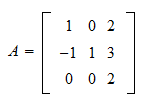as an example.

In WolframAlpha, this matrix is written as {{1,0,2},{-1,1,3},{0,0,2}}. Copy this string in the WolframAlpha search box and hit Return.Here is the result you get.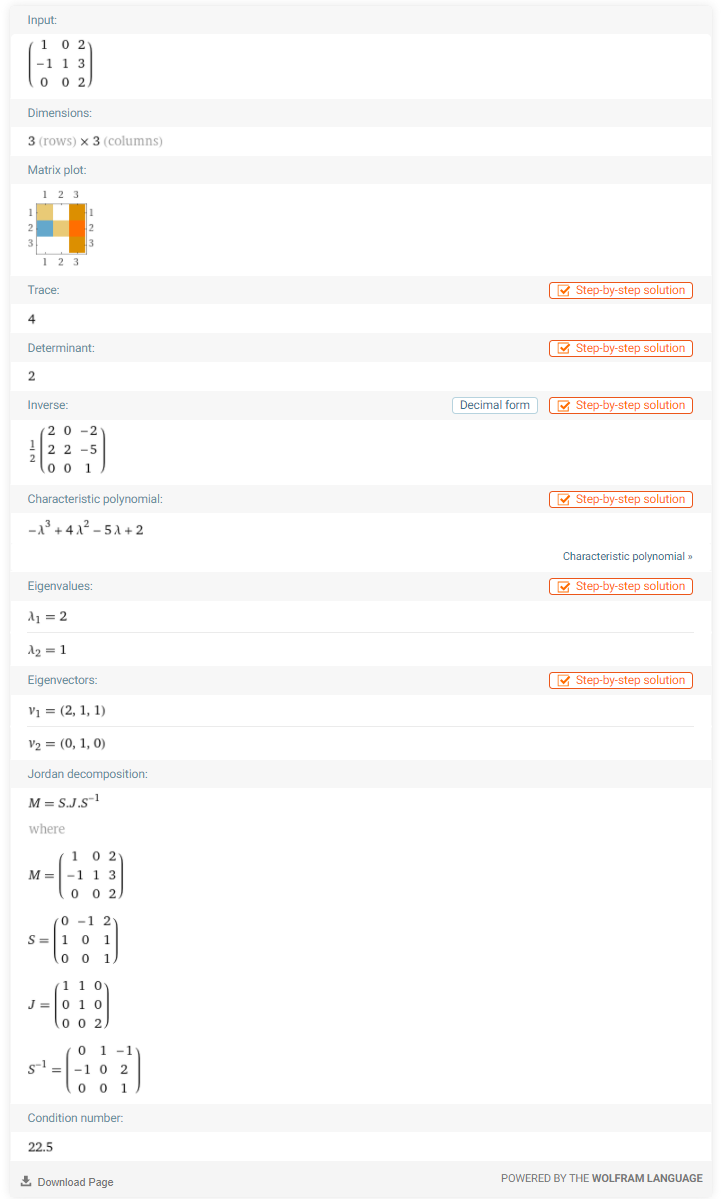As you can see, the matrix has two eigenvalues:and.

If you know about Jordan forms, you will immediately be able to see that the eigenvalueis repeated only once on the main diagonal of the Jordan form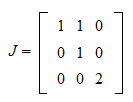Hence, both the algebraic and geometric multiplicity ofare equal to. Instead, the eigenvalueis repeated twice, which means that its algebraic multiplicity is equal to. Its geometric multiplicity is equal to the number of Jordan blocks associated to, which is equal to.

The general rule is: the geometric multiplicity of an eigenvalue is equal to the number of Jordan blocks associated to that eigenvalue.

Do not worry if you do not know about Jordan forms. In the next step, we are going to show how to find the geometric multiplicity without Jordan forms.

## Step 3: compute the RREF of the nilpotent matrix

Let us focus on the eigenvalue.

We know that an eigenvectorassociated toneeds to satisfywhereis theidentity matrix.

The eigenspace ofis the set of all such eigenvectors. Denote the eigenspace by. Then,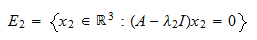The geometric multiplicity ofis the dimension of.

Note thatis the null space of.

By the rank-nullity theorem, the dimension ofmust be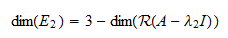whereis the range of.

In other words, the geometric multiplicitycan be found by calculating the dimension of the span of the columns of.

But this can be easily done by computing the reduced row echelon form (rref) of.

In WolframAlpha, the matrixis written as {{1,0,2},{-1,1,3},{0,0,2}} - IdentityMatrix.

To compute its rref, copy the following string in the search box and press Enter:

reduced row echelon form: ({{1,0,2},{-1,1,3},{0,0,2}} - IdentityMatrix)

Here is the result you get.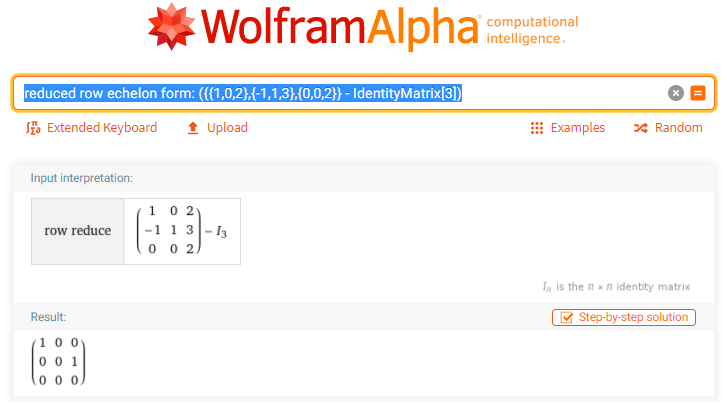As you can see, the rref ofhas two basic columns, which implies that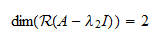As a consequence, the geometric multiplicity ofis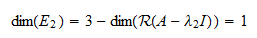## Step 4: show your appreciation

I guess we are done!